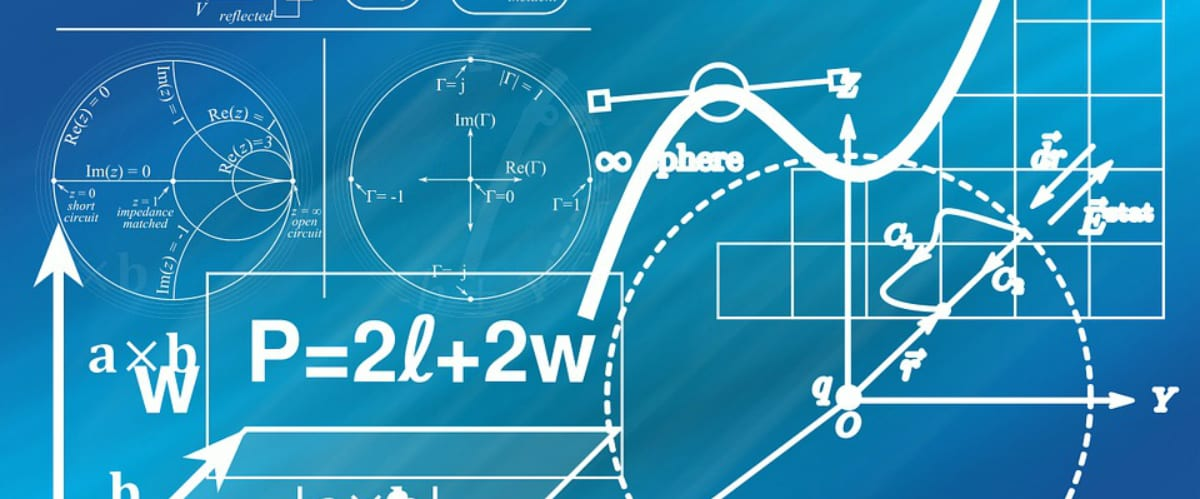# These 5 Math Riddles Are Designed to Challenge Even the Smartest Minds

Image Credit: Pixabay

Are you someone who is good at math? Or maybe you just think you are…

Either way, these 5 riddles are designed to challenge your skills, so give them a shot!

## #5. What is the lowest possible house number of two houses if the numbers are the reverse of each other and the difference ends in two?

19.

The two numbers would be 19 and 91.

## #4. How many eggs will half a dozen hens lay in half a dozen days?

2 dozen.

If you increase both the number of hens and the amount of time available four-fold, the number of eggs increases 16 times. 16 x 1.5 = 24.

## #3. How many cards are there?

There are 47 cards.

## #2. How many different 10-digit numbers can I type by moving my finger like a knight in chess?

You can form the numbers 5034927618 and 5038167294. You can also form their reverses: 8167294305 and 4927618305.

Hence four different numbers can be made.

The key point is to realize that the number must start or end on the ‘5’ key, followed/preceded by the ‘0’ key, otherwise, there is no way of using all ten keys during the route.